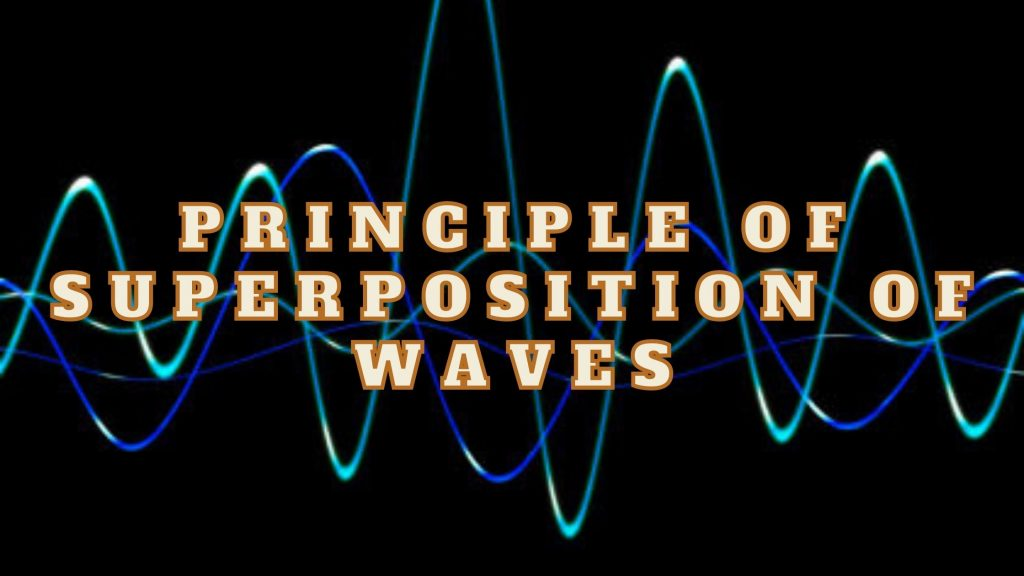Physics
Study Material

# Principle of Superposition of Waves- Sum of Amplitudes

When two or more waves travelling in same or opposite direction meet at a point, their resultant is algebraic sum of amplitudes. This is principle of superposition.

2 minutes long
Posted by Mahak Jain, 22/7/2021Hesap Oluştur

Got stuck on homework? Get your step-by-step solutions from real tutors in minutes! 24/7. Unlimited.

Have you ever wondered what happens when two wave pulses travelling in opposite directions cross each other? It turns out that wave pulses continue to retain their identities after they have crossed. But when they are at same position, they interfere with one other and resultant sum is algebraic sum of their amplitudes. This is known as principle of superposition of waves.

## Principle Statement of Superposition of Waves

According to this principle, each pulse moves as if others are not present. The constituents of the medium, therefore, suffer displacments due to both and since the displacements can be positive and negative, the net displacement is an algebraic sum of the two.

## Mathematical Derivation of Principle of Superposition

Let y1 (x,t) and y2 (x,t) be the displacements due to two wave disturbances in the medium. If the waves arrive in a region simultaneously, and therefore, overlap, the net displacement y (x,t) is

y(x,t) = y1(x,t) + y2(x,t)

Consider two harmonic travelling waves on a stretched string, both with the same ω (angular frequency) and k (wave number), and, therefore, the same wavelength λ. Their wave speed will be identical. Let us further assume that their amplitudes are equal and they are both travelling in the positive direction of x-axis. The waves only differ in their initial phase.

y1(x, t) = a sin (kx – ωt)

y2(x, t) = a sin (kx – ωt + φ)

The net displacement is then, by the principle of superposition, given by

y (x, t) = a sin (kx – ωt) + a sin (kx – ωt + φ)

Thus, final equation comes out to be y (x, t) = a cos (φ/2) sin (kx – ωt + φ/2)

This is also a harmonic travelling wave in the positive direction of x-axis, with the same frequency and wavelength. But there is a small catch that initial phase angle is φ/2.